# Support Vector Machine Algorithm

In this page we will learn about What is Support Vector Machine Algorithm in Machine Learning?, Types of SVM, Hyperplane and Support Vectors in the SVM algorithm, How does SVM works?, Python Implementation of Support Vector Machine, Fitting the SVM classifier to the training set.

## What is Support Vector Machine Algorithm?

The Support Vector Machine Algorithm, or SVM, is a popular Supervised Learning technique that may be used to solve both classification and regression issues. However, it is mostly utilized in Machine Learning for Classification difficulties.
The SVM algorithm's purpose is to find the optimum line or decision boundary for categorizing n-dimensional space into classes so that additional data points can be readily placed in the correct category in the future. A hyperplane is the name for the optimal choice boundary.
The extreme points/vectors that assist create the hyperplane are chosen via SVM. Support vectors are the extreme instances, and the algorithm is called a Support Vector Machine. Consider the diagram below, which shows how a decision boundary or hyperplane is used to classify two different categories: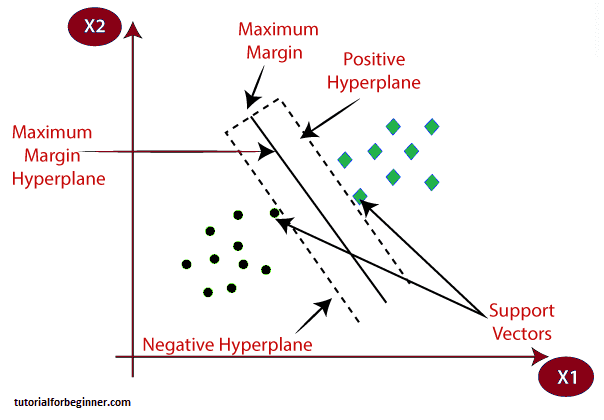Example: The example we used in the KNN classifier can help you understand SVM. If we observe an unusual cat that also has some dog-like characteristics, we can use the SVM method to develop a model that can properly identify whether it is a cat or a dog. We'll first train our model with a large number of photographs of cats and dogs so that it can learn about their many characteristics, and then we'll put it to the test with this weird creature. As a result, the extreme case of cat and dog will be shown because the support vector forms a decision boundary between these two data (cat and dog) and chooses extreme cases (support vectors). It will be classified as a cat based on the support vectors.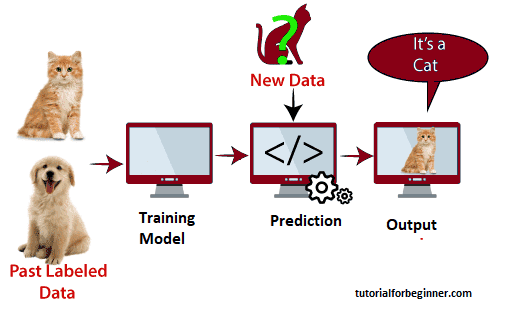Face detection, image classification, text categorization, and other tasks can all benefit from the SVM method.

## Types of SVM

SVM can be of two types:

• Linear SVM: Linear SVM is a classifier that is used for linearly separable data, which implies that if a dataset can be classified into two classes using a single straight line, it is called linearly separable data, and the classifier is named Linear SVM.
• Non-linear SVM: Non-linear SVM is used for non-linearly separated data, which means that if a dataset cannot be classified using a straight line, it is non-linear data, and the classifier used is Non-linear SVM

## Hyperplane and Support Vectors in the SVM algorithm:

In n-dimensional space, there can be several lines/decision boundaries to separate the classes, but we must choose the optimum decision boundary to help classify the data points. The hyperplane of SVM refers to the best boundary.
The hyperplane's dimensions are determined by the features in the dataset; for example, if there are two features (as shown in the image), the hyperplane will be a straight line. If three characteristics are present, the hyperplane will be a two-dimensional plane.
We always make a hyperplane with a maximum margin, which refers to the distance between data points.

## Support Vector:

Support Vectors are the data points or vectors that are closest to the hyperplane and have an effect on the hyperplane's position. These vectors are called Support vectors because they support the hyperplane.

## How does SVM works?

### Linear SVM:

An example can be used to explain how the SVM algorithm works. Assume that we have a dataset with two tags (green and blue) and two features (x1 and x2). We're looking for a classifier that can categorize the pair of coordinates (x1, x2) as green or blue. Consider the following illustration:
An example can be used to explain how the SVM algorithm works. Assume that we have a dataset with two tags (green and blue) and two features (x1 and x2). We're looking for a classifier that can categorize the pair of coordinates (x1, x2) as green or blue. Consider the following illustration: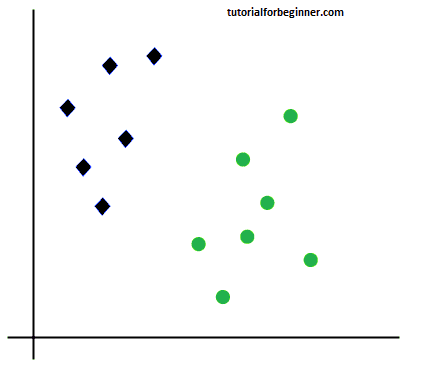Because this is a two-dimensional space, we can easily separate these two classes by drawing a straight line between them. However, numerous lines can be used to divide these classes. Consider the following illustration: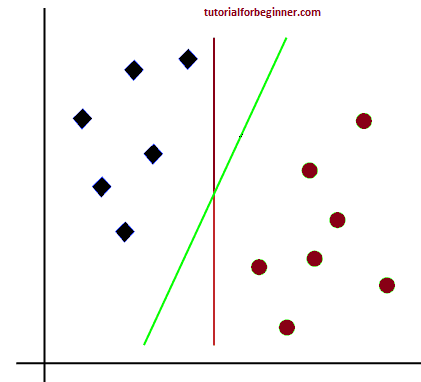As a result, the SVM method aids in the discovery of the best line or decision boundary, which is referred to as a hyperplane. The SVM method locates the intersection of the lines from both classes. Support vectors are the names given to these points. Margin is the distance between the vectors and the hyperplane. The purpose of SVM is to increase this margin as much as possible. The ideal hyperplane is the one with the greatest margin.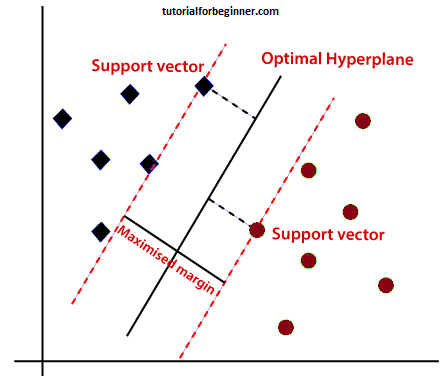## Non-Linear SVM:

We can separate data that is linearly structured using a straight line, but we cannot draw a single straight line for non-linear data. Consider the following illustration: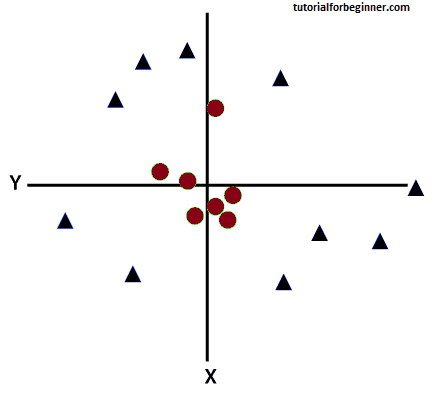So we'll need to add another dimension to distinguish these data pieces. We've utilized two dimensions for linear data, x and y, so we'll add a third dimension, z, for non-linear data. It can be calculated using the following formula:

### z = x2 + y2

The example space will look like this after adding the third dimension: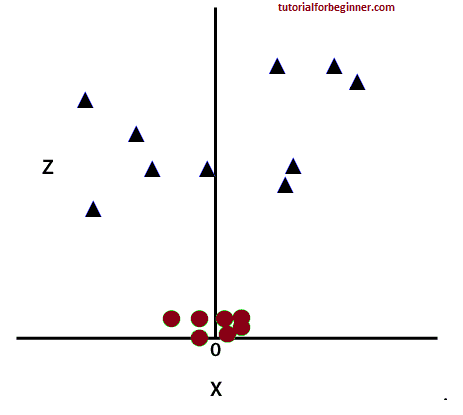So now, SVM will divide the datasets into classes in the following way. Consider the below image: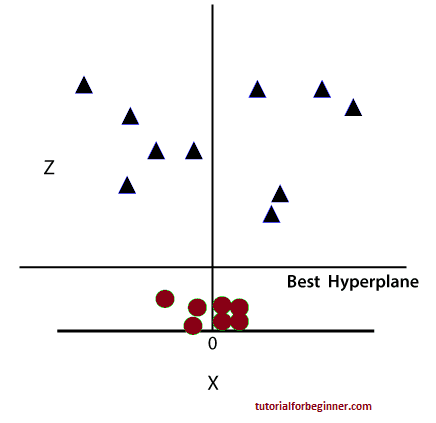Because we are in 3-d space, it appears to be a plane parallel to the x-axis. If we convert it to 2d space with z=1, it looks like this: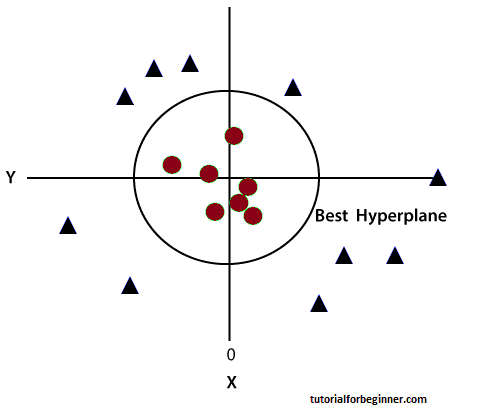In the case of non-linear data, we get a circumference of radius 1.

## Python Implementation of Support Vector Machine

Now we'll use Python to develop the SVM algorithm. We'll use the same dataset user data that we used for KNN classification and Logistic regression.

## Data Pre-processing step

The code will remain the same till the Data pre-processing stage. The code is as follows:

``````
#Data Pre-processing Step
# importing libraries
import numpy as nm
import matplotlib.pyplot as mtp
import pandas as pd

d#importing datasets

#Extracting Independent and dependent Variable
x = data_set.iloc[:, [2,3]].values
y = data_set.iloc[:, 4].values

#Splitting the dataset into training and test set.
from sklearn.model_selection import train_test_split
x_train, x_test, y_train, y_test = train_test_split(x, y, test_size = 0.25, random_state = 0)
#feature Scaling
from sklearn.preprocessing import StandardScaler
st_x = StandardScaler()
x_train = st_x.fit_transform(x_train)
x_test = st_x.transform(x_test)
```
```

We will pre-process the data after executing the above code. The code will give the dataset as: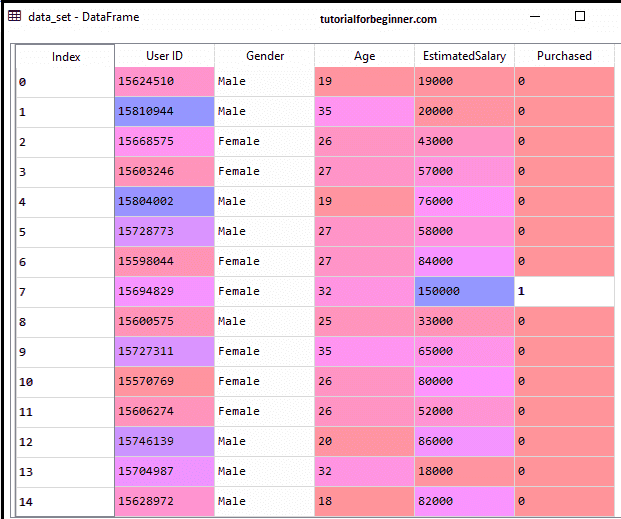The scaled output for the test set will be: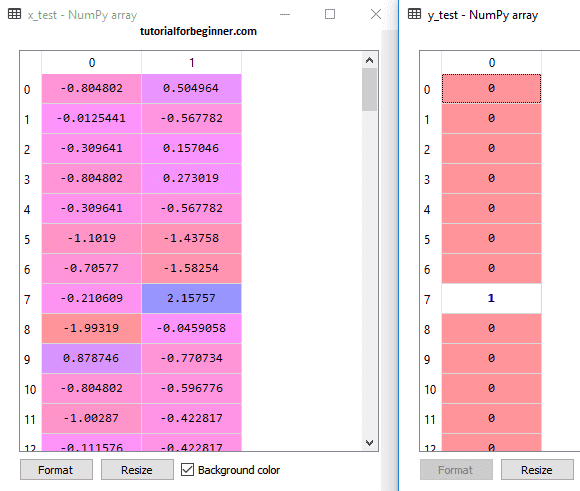## Fitting the SVM classifier to the training set:

The SVM classifier will now be fitted to the training set. We'll use the SVC class from the Sklearn.svm package to build the SVM classifier. The code for it is as follows:

``````
#"Support vector classifier"
rom sklearn.svm import SVC
classifier = SVC(kernel='linear', random_state=0)
classifier.fit(x_train, y_train)
```
```

We used kernel='linear' in the above code since we are generating SVM for linearly separable data. For non-linear data, though, we can adjust it. The classifier was then fitted to the training dataset (x train, y train).

## Output:

```
```
Out:
SVC(C = 1.0, cache_size = 200, class_weight = None, coef0 = 0.0,
decision_function_shape = 'ovr', degree =3,
gamma = 'auto_deprecated',
kernel = 'linear', max_iter = -1, probability = False,
random_state=0,
shrinking = True, tol = 0.001, verbose = False)
```
```

Change the values of C(Regularization factor), gamma, and kernel to vary the model's performance.

## Predicting the test set result:

We'll now predict the output for the test set. We'll do this by creating a new vector called y pred. The code for I is shown below.

``````
#Predicting the test set result
y_pred = classifier.predict(x_test)
```
```

After getting the y pred vector, we can compare the y pred and y test results to see how much the actual value differs from the anticipated value.

## Output:

The following is the output for the test set prediction: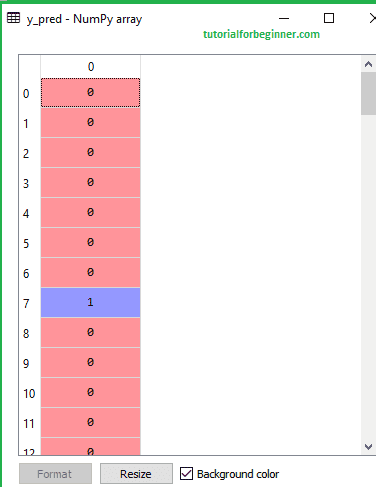Creating the confusion matrix: Now we'll compare the performance of the SVM classifier to the Logistic regression classifier to see how many false predictions it makes. To make the confusion matrix, we'll need to use the sklearn library's confusion matrix function. We'll use a new variable cm to call the function when it's been imported. The function has two parameters: y true (the actual values) and y pred (the predicted values) (the targeted value return by the classifier). The code for it is as follows:

``````
#Creating the Confusion matrix
from sklearn.metrics import confusion_matrix
cm = confusion_matrix(y_test, y_pred)
```
```

## Output: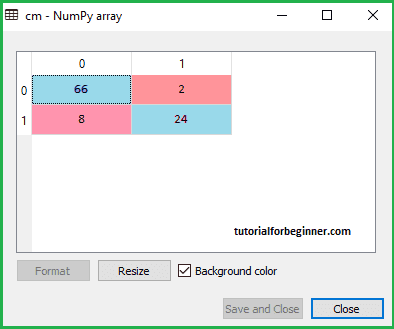There are 66+24= 90 correct predictions and 8+2= 10 correct predictions, as seen in the above output image. As a result, we can say that our SVM model outperformed the Logistic regression model.

## Visualizing the training set result:

The result of the training set will now be visualized; the code for this may be seen below:

``````
from matplotlib.colors import ListedColormap
x_set, y_set = x_train, y_train
x1, x2 = nm.meshgrid(nm.arange(start = x_set[:, 0].min() - 1, stop = x_set[:, 0].max() + 1, step = 0.01),
nm.arange(start = x_set[:, 1].min() - 1, stop = x_set[:, 1].max() + 1, step = 0.01))
mtp.contourf(x1, x2, classifier.predict(nm.array([x1.ravel(), x2.ravel()]).T).reshape(x1.shape),
alpha = 0.75, cmap = ListedColormap(('red', 'green')))
mtp.xlim(x1.min(), x1.max())
mtp.ylim(x2.min(), x2.max())
for i, j in enumerate(nm.unique(y_set)):
mtp.scatter(x_set[y_set == j, 0], x_set[y_set == j, 1],
c = ListedColormap(('red', 'green'))(i), label = j)
mtp.title('SVM classifier (Training set)')
mtp.xlabel('Age')
mtp.ylabel('Estimated Salary')
mtp.legend()
mtp.show()
```
```

## Output:

By executing the above code, we will get the output as: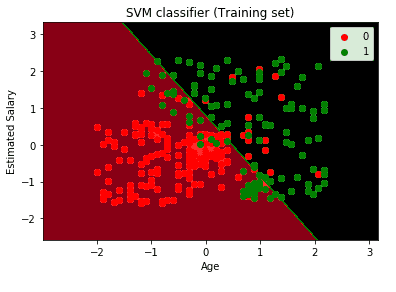As can be seen, the above output looks a lot like the Logistic regression output. We received the straight line as hyperplane in the output since we utilized a linear kernel in the classifier. We've already shown that the hyperplane in SVM is a straight line in 2d space.

## Visualizing the test set result:

``````
#Visulaizing the test set result
from matplotlib.colors import ListedColormap
x_set, y_set = x_test, y_test
x1, x2 = nm.meshgrid(nm.arange(start = x_set[:, 0].min() - 1, stop = x_set[:, 0].max() + 1, step  = 0.01),
nm.arange(start = x_set[:, 1].min() - 1, stop = x_set[:, 1].max() + 1, step = 0.01))
mtp.contourf(x1, x2, classifier.predict(nm.array([x1.ravel(), x2.ravel()]).T).reshape(x1.shape),
alpha = 0.75, cmap = ListedColormap(('red','green' )))
mtp.xlim(x1.min(), x1.max())
mtp.ylim(x2.min(), x2.max())
for i, j in enumerate(nm.unique(y_set)):
mtp.scatter(x_set[y_set == j, 0], x_set[y_set == j, 1],
c = ListedColormap(('red', 'green'))(i), label = j)
mtp.title('SVM classifier (Test set)')
mtp.xlabel('Age')
mtp.ylabel('Estimated Salary')
mtp.legend()
mtp.show()
```
```

## Output:

By executing the above code, we will get the output as: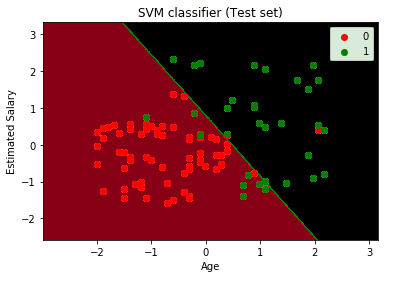The SVM classifier has separated the users into two zones, as shown in the above output image (Purchased or Not purchased). Users who bought the SUV are represented by the red scatter spots. Users who did not purchase the SUV are represented by green scatter points in the green region. The hyperplane has separated the two classes into variables that have been purchased and variables that have not been purchased.